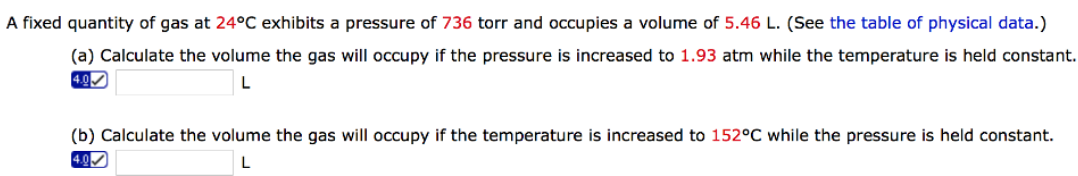# Problem: A fixed quantity of gas at 24°C exhibits a pressure of 736 torr and occupies a volume of 5.46 L. (See the table of physical data.) (a) Calculate the volume the gas will occupy if the pressure is increased to 1.93 atm while the temperature is held constant. (b) Calculate the volume the gas will occupy if the temperature is increased to 152°C while the pressure is held constant.

###### FREE Expert Solution###### Problem Details

A fixed quantity of gas at 24°C exhibits a pressure of 736 torr and occupies a volume of 5.46 L. (See the table of physical data.)

(a) Calculate the volume the gas will occupy if the pressure is increased to 1.93 atm while the temperature is held constant.

(b) Calculate the volume the gas will occupy if the temperature is increased to 152°C while the pressure is held constant.